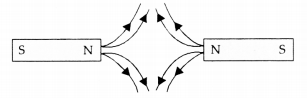# Magnetic lines of force of two pairs of magnets are shown in figure A and B, Out of these two figures

Magnetic lines of force of two pairs of magnets are shown in figure A and B, Out of these two figures, which one represents the correct pattern of field lines. Name the poles of magnets facing each other.

Figure B represents the correct pattern of field lines of pair of magnets. Figure A does not represent the correct pattern of field lines because field lines never cross each other.Poles of magnets facing each other are north poles as the field lines emerge from a magnet at N-pole.